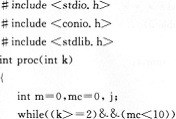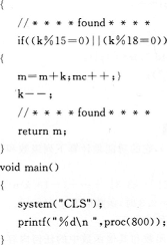题目内容 （请给出正确答案）

# 下列程序的功能是：选出5000以下符合条件的自然数。条件...

include＜stdio.h＞

int cnt,sum;

void countValue()

{

}

void main()

{

cnt=sum=0;

countValue ();

printf ("满足条件的自然数的个数=%d\n", cnt);

printf ("满足条件的自然的值的和=%d\n", sum);

writeDAT ();

}

writeDAT ()

{

FILE *fp;

fp=fopen ("OUT67. DAT" , "w" );

fprintf (fp, "%d\n%d\n", cnt, sum);

fclose {fp);

}更多“下列程序的功能是：选出5000以下符合条件的自然数。条件..”相关的问题

include＜stdio. h＞
int cnt, sum;
void countValue()
{
}
void main ()
{
cnt=sum=O;
countValue ();
printf ("满足条件的个数=%d\n", cnt);
printf ("满足条件所有的SIX与NINE的和=%d\n", sum);
writeDAT ();
}
writeDAT ( )
{
FILE *fp;
fp=fopen("OUT51.DAT", "w");
fprintf (fp, "%d\n%d\n", cnt, sum);
fclose (fp);
}Private Sub Command1_Click() Dim x As Long， y As Long，i As Long x=Val(Text1．Text) y=Val(TextText) TextText = Str(【 】) End Sub Function fun1(a As Long，b As Long)As Long Dim r As Long Dim t As Long If【 】Then t = a a = b b = t End If r = a Mod b Do While r ＜＞ 0 a = b b = r r = a Mod b Loop 【 】 End FunctionPrivate Sub Command1_Click()
Dim n As Long
Dim m As Long
Dim s As Single
Dim d As String
For n=1 To 30
For m=1 To【 】
s =n+ m
【 】
If Sqr (s)=Int(Sqr(s))【 】Sqr(D)=Int(Sqr(D))Then
Print n：m
End lf
Next m
Next n
End SubPrivate Sub Command1_Click()
Dim n As Long
Dim m As Long
Dim s As Single
Dim d As String
For n=1 To 30
For m=1 To【 】
s =n+ m
【 】
If Sqr (s)=Int(Sqr(s))【 】Sqr(D)=Int(Sqr(D))Then
Print n：m
End lf
Next m
Next n
End Sub如搜索结果不匹配，请 联系老师上传试题 获取答案微信搜一搜上学吧微信搜一搜上学吧